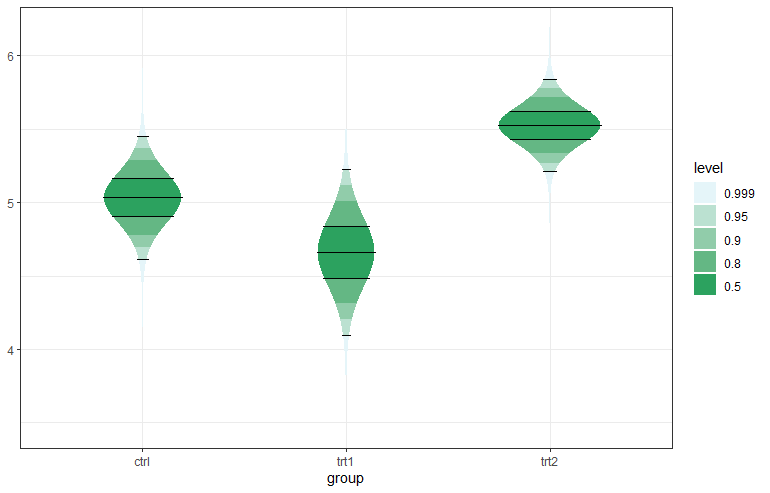# ggstudent - Continuous Confidence Interval Plots using t-Distribution

The R package ggstudent provides an extension to `ggplot2` for creating two types of continuous confidence interval plots (Violin CI and Gradient CI plots), typically for the sample mean. These plots contain multiple user-defined confidence areas with varying colours, defined by the underlying t-distribution used to compute standard confidence intervals for the mean of the normal distribution when the variance is unknown.

Two types of plots are available, a gradient plot with rectangular areas, and a violin plot where the shape (horizontal width) is defined by the probability density function of the t-distribution.

Here is an example:

``````library("magrittr")
library("dplyr")
library("ggplot2")
library("scales")

ci_levels <- c(0.999, 0.95, 0.9, 0.8, 0.5)
n <- length(ci_levels)
ci_levels <- factor(ci_levels, levels = ci_levels)
PlantGrowth %>% dplyr::group_by(group) %>%
dplyr::summarise(
mean = mean(weight),
df = dplyr::n() - 1,
se = sd(weight)/sqrt(df + 1)) %>%
dplyr::full_join(
data.frame(group =
rep(levels(PlantGrowth\$group), each = n),
level = ci_levels), by = "group") -> d

p <- ggplot(data = d, aes(group)) +
geom_student(aes(mean = mean, se = se, df = df,
level = level, fill = level), draw_lines = c(0.95, 0.5))